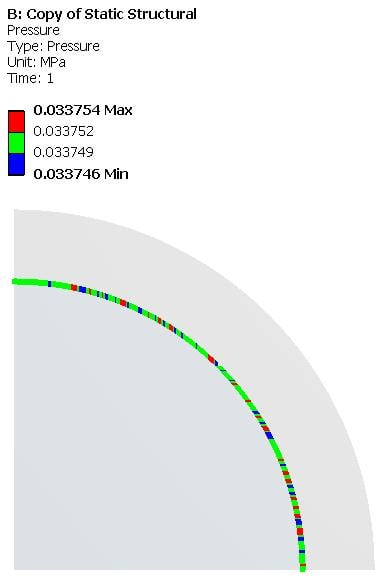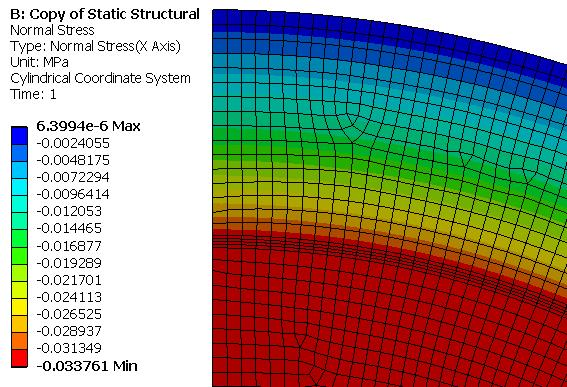peteroznewman
Subscriber

1) You should use at least 4 elements through the thickness of the ring sleeve. One element is inadequate.

2) If you make a Cylindrical Coordinate System then the X-axis will be radial and you will get a more uniform display. With the Global coordinate system, you get a high point along the X axis and a minimum point 90 degrees around the circumference.  You don't need a cylindrical coordinate system if you insert a Contact Tool in the Solution Branch and Insert a Pressure result.

3) The equation is for either plane stress or plane strain, which you can get in a 2D model. Do you know what were the assumptions in that equation?  If you make a 2D model, the surfaces must be in the XY plane and you must set the Geometry cell Property of Analysis Type to 2D in the Workbench interface before you transfer that geometry to Mechanical when you open the Model cell.

4) It will be better if you slice the model with 2 planes to make a 1/4 pie slice. Use a displacement BC to set just one displacement, the normal to the face, equal to zero.  This is true for both 2D and 3D.

5) The penetration in the contact is less using Normal Lagrange than Augmented Lagrange.

6) Your calculation of the expected Normal Stress (Pressure) is off by a factor of 1000.  The answer I got using the formula above and values you provided is 0.03375 MPa

7) If I make a model using the methods I outline above, using Plane Stress equations, I get a Normal Stress of 0.03375 MPa, which is in near perfect agreement with the equation.  If I switch to Plane Strain equations, the Normal Stress is 0.0371 MPa.